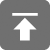# 工学1号馆

home

## Project Euler 17–Number letter counts

Wu Yudong    August 11, 2018     欧拉计划   576

If the numbers 1 to 5 are written out in words: one, two, three, four, five, then there are 3 + 3 + 5 + 4 + 4 = 19 letters used in total.

If all the numbers from 1 to 1000 (one thousand) inclusive were written out in words, how many letters would be used?

NOTE: Do not count spaces or hyphens. For example, 342 (three hundred and forty-two) contains 23 letters and 115 (one hundred and fifteen) contains 20 letters. The use of “and” when writing out numbers is in compliance with British usage.

#include <stdio.h>
#include <stdbool.h>

int a = {0,3,3,5,4,4,3,5,5,4,3,6,6,8,8,7,7,9,8,8};

void init(void)  //初始化数组
{
a = 6;
a = 6;
a = 5;
a = 5;
a = 5;
a = 7;
a = 6;
a = 6;
a = 7;
}

int within100(void)  //计算1~99所含字母的和
{
int i, sum, t;
t = sum = 0;
for(i = 1; i <= 9; i++) t += a[i];
for(i = 1; i <= 19; i++) sum += a[i];
for(i = 2; i <= 9; i++) {
sum += a[i*10] * 10;
sum += t;
}
return sum;
}

void solve(void)
{
int i;
int sum, t;
sum = t = within100();
for(i = 1; i < 10; i++) {
sum += (a[i] + 10) * 99 + (a[i] + 7) + t;
}
sum += 11;

printf("%d\n",sum);
}

int main(void)
{
init();
solve();
return 0;
}